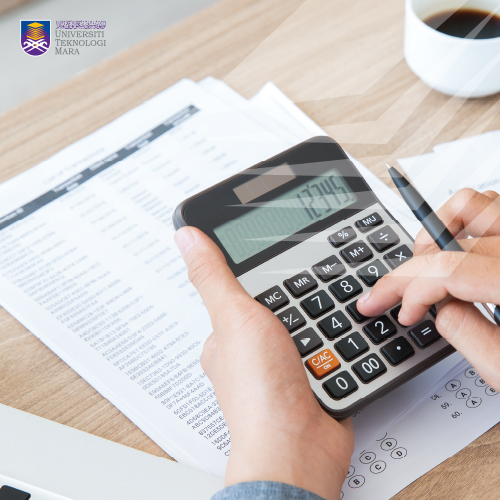• We are available for any custom works this month
• Main office: Springville center X264, Park Ave S.01
• Call us (123) 456-7890 - (123) 555-7891

## Course Information

### BIOSTATISTICS AND CALCULUS

•••This course is aimed to equip students with the basic knowledge in statistics necessary in the pharmaceutical area. The topics covered are related to basic concept of statistics, epidemiology statistics and basic introduction on SPSS software for analysing data.

#### Course Syllabus

Introduction to the course and Basic Statistics
1. Population and Sample
2. Types of Data/Variables
3. Scale of Measurements
4. Frequency Distribution
5. Measures of Central Tendency
6. Measures of Dispersion

Probability Distribution
1. Discrete Probability Distributions
2. The Binomial Distribution
3. The Poisson Distribution
4. Continuous Probability Distributions
5. The Normal Distribution
6. Normal Distribution Applications

Sampling Distributions and Hypothesis Testing
1. Sample Mean Distribution
2. Sample Proportion Distribution
3. Critical Region and Critical Values
4. One-tailed and Two-tailed tests
5. Type I error and Type II errors

Parametric Statistical Techniques
1. T-tests
2. One-way ANOVA

Nonparametric Statistical Techniques
1. Signed Test
2. Wilcoxon-Signed Rank Test
3. Mann-Whitney Test
4. Kruskal-Wallis Test

Correlation
1. Types of Correlation
2. Pearson?s Product Moment Correlation Coefficient
3. Spearman?s Rank Correlation

Regression
1. Linear Least-Squares Regression

An Overview of Epidemiology Statistics
1. Measures of disease frequency and rate
2. Study designs
3. Health Analytics

Calculus Part 1: Differentiation
1. Concepts of limits & the derivative
2. Basic Rules of differentiation
3. Application of the derivative

Calculus Part 2: Integration
1. Concepts of indefinite integral
2. Basic rules of integration
3. Concepts of area & definite integral
4. Application of definite integral

SPSS: Introduction to SPSS
1. Getting started to SPSS
2. Preparing the data file (incl. negatively worded data)
3. Preliminary analyses

SPSS: Statistical Techniques (Relationship between Variables)
1. Chi-square for independence
2. Correlation
3. Regression analysis

SPSS: Statistical Techniques (Parametric)
1. T-tests
2. One-way ANOVA

SPSS: Statistical Techniques (Nonparametric)
1. Signed Test
2. Wilcoxon-Signed Rank Test
3. Mann-Whitney Test
4. Kruskal-Wallis Test

SPSS: Statistical Techniques (Reliability & Factor Analysis)
1. Reliability analysis
2. Assumption testing
3. Factor analysis